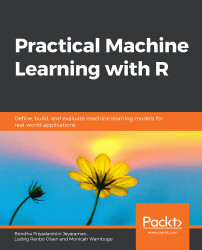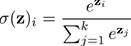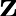•#### Practical Machine Learning with R#### Overview of this book

With huge amounts of data being generated every moment, businesses need applications that apply complex mathematical calculations to data repeatedly and at speed. With machine learning techniques and R, you can easily develop these kinds of applications in an efficient way. Practical Machine Learning with R begins by helping you grasp the basics of machine learning methods, while also highlighting how and why they work. You will understand how to get these algorithms to work in practice, rather than focusing on mathematical derivations. As you progress from one chapter to another, you will gain hands-on experience of building a machine learning solution in R. Next, using R packages such as rpart, random forest, and multiple imputation by chained equations (MICE), you will learn to implement algorithms including neural net classifier, decision trees, and linear and non-linear regression. As you progress through the book, you’ll delve into various machine learning techniques for both supervised and unsupervised learning approaches. In addition to this, you’ll gain insights into partitioning the datasets and mechanisms to evaluate the results from each model and be able to compare them. By the end of this book, you will have gained expertise in solving your business problems, starting by forming a good problem statement, selecting the most appropriate model to solve your problem, and then ensuring that you do not overtrain it.Free Chapter
An Introduction to Machine LearningData Cleaning and Pre-processingFeature EngineeringIntroduction to neuralnet and Evaluation MethodsLinear and Logistic Regression ModelsUnsupervised LearningAppendix## Multiclass Classification Overview

When we have more than two classes, we have to modify our approach slightly. In the output layer of the neural network, we now have the same number of nodes as the number of classes. The values in these nodes are normalized using the softmax function, such that they all add up to 1. We can interpret these normalized values as probabilities, and the node with the highest probability is our predicted class. The softmax function is given by, whereis the vector of output nodes.

When evaluating the model, we have to increase the size of our confusion matrix. Figure 4.16 shows a confusion matrix with three classes. The "Yay!" boxes contain the counts of correct predictions, while the "Nope!" boxes contain the counts of incorrect predictions:

###### Figure 4.16: The confusion matrix with three classes

With this, we can calculate both overall metrics and one-vs-all metrics. In one-vs-all evaluations, we have one class (such as class...